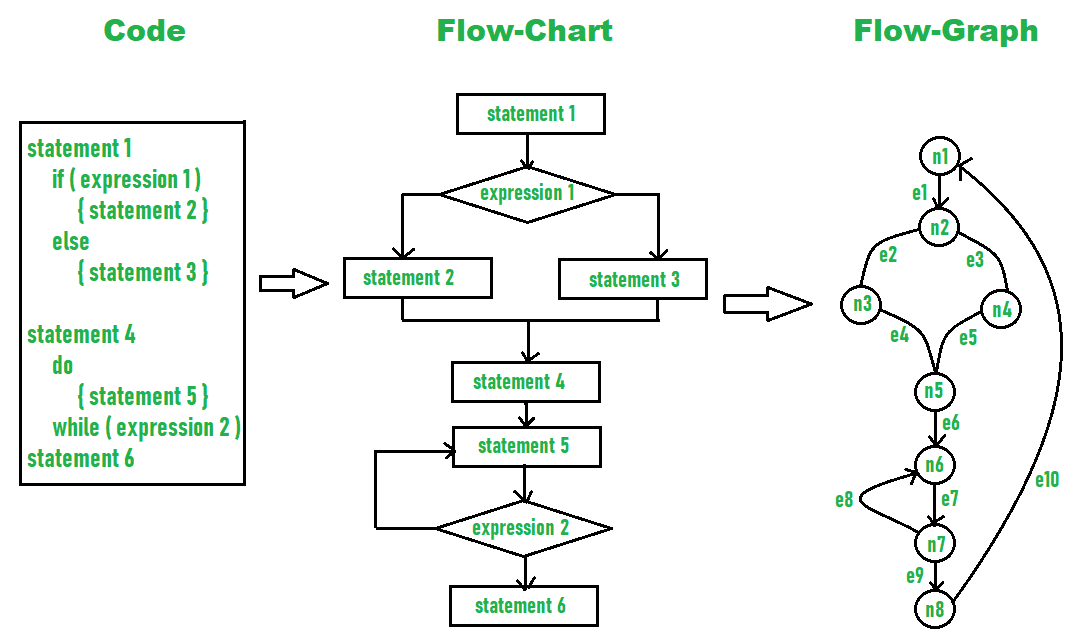# Metrics for the Design Model of the Product

Metrics simply measures quantitative assessment that focuses on countable values most commonly used for comparing and tracking performance of system. Metrics are used in different scenarios like analyzing model, design model, source code, testing, and maintenance. Metrics for design modeling allows developers or software engineers to evaluate or estimate quality of design and include various architecture and component-level designs.

Metrics by Glass and Card :
In designing a product, it is very important to have efficient management of complexity. Complexity itself means very difficult to understand. We know that systems are generally complex as they have many interconnected components that make it difficult to understand. Glass and Card are two scientists who have suggested three design complexity measures. These are given below :

1. Structural Complexity –
Structural complexity depends upon fan-out for modules. It can be defined as :

`S(k) = f2out(k)`

Where fout represents fanout for module k (fan-out means number of modules that are subordinating module k).

2. Data Complexity –
Data complexity is complexity within interface of internal module. It is size and intricacy of data. For some module k, it can be defined as :

`D(k) = tot_var(k) / [fout(k)+1]`

Where tot_var is total number of input and output variables going to and coming out of module.

3. System Complexity –
System complexity is combination of structural and data complexity. It can be denoted as:

`Sy(k) = S(k)+D(k)`

When structural, data, and system complexity get increased, overall architectural complexity also gets increased.

Complexity metrics –
Complexity metrics are used to measure complexity of overall software. The computation if complexity metrics can be done with help of a flow graph. It is sometimes called cyclomatic complexity. The cyclomatic complexity is a useful metric to indicate complexity of software system. Without use of complexity metrics, it is very difficult and time-consuming to determine complexity in designing products where risk cost emanates. Even continuous complexity analysis makes it difficult for project team and management to solve problem. Measuring Software complexity leads to improve code quality, increase productivity, meet architectural standards, reduce overall cost, increases robustness, etc. To calculate cyclomatic complexity, following equation is used:

`Cyclomatic complexity= E - N + 2 `

Where, E is total number of edges and N is total number of nodes.

Example –
In diagram given below, you can see number of edges and number of nodes.So, the Cyclomatic complexity can be calculated as –

```Given,
E = 10,
N = 8

So,
Cyclomatic complexity
= E - N + 2
= 10 – 8 + 2
= 4 ```

Attention reader! Don’t stop learning now. Get hold of all the important CS Theory concepts for SDE interviews with the CS Theory Course at a student-friendly price and become industry ready.

My Personal Notes arrow_drop_upCheck out this Author's contributed articles.

If you like GeeksforGeeks and would like to contribute, you can also write an article using contribute.geeksforgeeks.org or mail your article to contribute@geeksforgeeks.org. See your article appearing on the GeeksforGeeks main page and help other Geeks.

Please Improve this article if you find anything incorrect by clicking on the "Improve Article" button below.

Article Tags :

Be the First to upvote.

Please write to us at contribute@geeksforgeeks.org to report any issue with the above content.